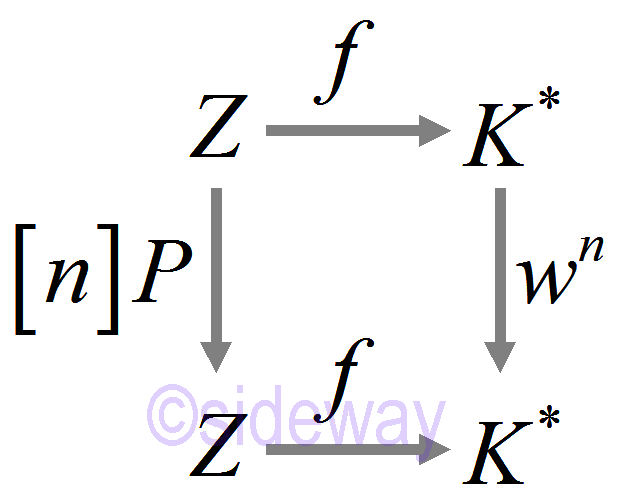output.to from Sideway
Draft for Information Only

# Content

`Pythagorean Triples Example 𝑥3+2𝑦3=3 Source and Reference`

# Pythagorean Triples

Binary operation on 𝑥2+𝑑𝑦2=1⇒(𝑥,𝑦)⊕(𝑤,𝑧)=(𝑥𝑧−𝑑𝑦𝑤,𝑥𝑤+𝑦𝑧) Consider 𝑥2+𝑑𝑦2=1 over a finite field 𝔽𝑝 Theorem: Wherther or not 𝑑 is a square in 𝔽𝑝, there is a solution 𝑃 such that 𝑍 is generated by 𝑃, i.e. 𝑄=𝑃⊕⋯⊕𝑃 for all 𝑄∊𝑍
This single generator is similar to the primitive root mod 𝑃
Proof: Consider the field ext 𝐾=𝔽𝑝(𝑑). Denote: [𝑛]𝑃=𝑃⊕⋯⊕𝑃. Define 𝑓:𝑍→𝐾 by 𝑓(𝑄)=𝑥+𝑦𝑑 with rational solution (𝑥,𝑦).Existence of a primitive root: 𝑔∊𝐾 s.t. {𝑔𝑛: 𝑛=0, 1, ⋯}=𝐾*. □ Fact: A subset of finitely many solutions will not generate 𝑍 under the operation. Key ingredients for parameterisation: 𝑥2+𝑑𝑦2=1
• A rational point (1,0)
• The degree being 2⇒𝑚−1: 𝑄→𝑍0
No easy way to generalize the operation for 𝑥3+𝑦3=1 ⇒(𝑥+𝑦)(𝑥+𝑦𝜁)(𝑥+𝑦𝜁2)=1, where 𝜁3=1 and 𝜁2+𝜁+1=0 ⇒(𝑥+𝑦)𝑛(𝑥+𝑦𝜁)𝑛(𝑥+𝑦𝜁2)𝑛=1 Fact: Let 𝑍={(𝑥,𝑦):𝑥3+𝑦3=1} The existence of Φ:𝑄→𝑍 contradicts some genus formula especially some positive. That is the topological invariant to the complex solution of the cubic curve⇒no parameterization.
Idea: One rational point and a tangent line → given a rational point.
Intesection to degree 2 such that a cubic curve of degree 3 to degree 2 and the tangent line with

## Example 𝑥3+2𝑦3=3

𝑍={𝑥3+2𝑦3=3}. 𝑃=(1,1)∊𝑍. Let 𝐹(𝑥,𝑦)=𝑥3+2𝑦3−3 tangent line at (𝑎,𝑏)=∂𝐹∂𝑥(𝑥−𝑎)+∂𝐹∂𝑦(𝑦−𝑏)=3𝑎2(𝑥−𝑎)+6𝑏2(𝑦−𝑏)=0 So 3(𝑥−1)+6(𝑦−1)=0 and 𝑥3+2𝑦3=3 subst. 𝑧=𝑥−1, 𝑤=𝑦−1 3𝑧+6𝑤=0 and (𝑧+1)3+2(𝑤+1)3=3 6𝑤2(3−𝑤)=0⇒𝑤=3⇒𝑦=4, 𝑥=−5 Idea: repeat with (−5,4) Line: 25(𝑥+5)+32(𝑦−4)=0 and 𝑥3+2𝑦3=3 subst. 𝑧=𝑥+5, 𝑤=𝑦−4 𝑥=655/253, 𝑦=−488/253, 253=11*23 Therefore (1,1)→(−5,4)→(655/253,−488/253)
Idea: Two rational points and a secant line→a rational point
741253(𝑥−1)−402253(𝑦−1)=0 and 𝑥3+2𝑦3=3 subst. 𝑧=𝑥−1, 𝑤=𝑦−1 741𝑧+402𝑤=0 and (𝑧+1)3+2(𝑤+1)3=3 27732342𝑤315069223+419922𝑤261009+1080𝑤247=0 Therefore 𝑤→0, −741253(𝑥−1)=0⇒𝑤𝑤+741253=0⇒a factor of cubic equation By long division ⇒𝑤𝑤+74125327732342𝑤15069223+9108061009=0 ⇒𝑥=2630918269, 𝑦=344918269⇒𝑥≈1.44, 𝑦≈0.18 Tangent line, 𝑃⊕𝑄 and Secant line, 𝑃 ⇒𝑅 Mordell-Weil Theorem: There are a finite subset of solutions that generate all solutions.

## Source and Reference

ID: 201100017 Last Updated: 11/17/2020 Revision: 0 Ref:References

1. B. Joseph, 1978, University Mathematics: A Textbook for Students of Science &amp; Engineering
2. Wheatstone, C., 1854, On the Formation of Powers from Arithmetical Progressions
3. Stroud, K.A., 2001, Engineering Mathematics
4. Coolidge, J.L., 1949, The Story of The Binomial TheoremHome 5

Management

HBR 3

Information

Recreation

Culture

Chinese 1097

English 337

Computer

Hardware 153

Software

Application 206

Latex 35

Manim 172

Numeric 19

Programming

Web 285

Unicode 504

HTML 65

CSS 59

ASP.NET 196

OS 422

Python 61

Knowledge

Mathematics

Algebra 31

Geometry 21

Calculus 67

Engineering

Mechanical

Rigid Bodies

Statics 92

Dynamics 37

Control

Natural Sciences

Electric 27